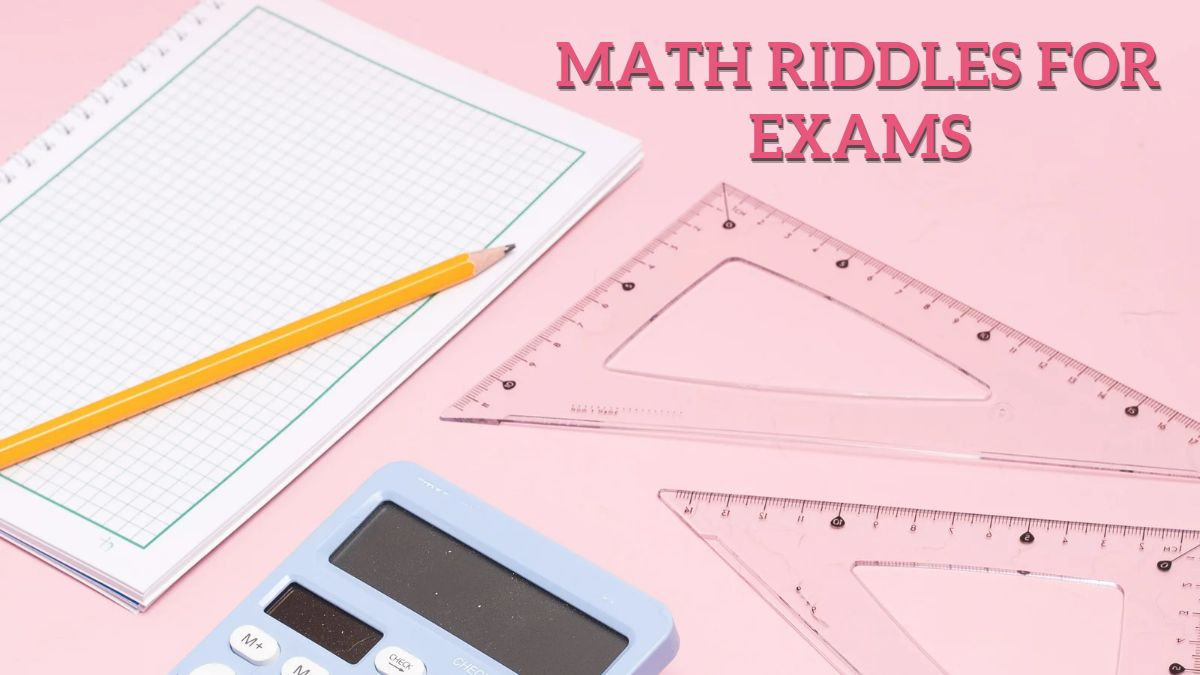# Math Riddles: 5 Questions On Calculating Area And Perimeter

These math riddles on area and perimeter are to help you prepare for your exam, be it school or competitive. Test your math skills now with these math riddles.Math Riddles: 5 Questions On Calculating Area And Perimeter

Are you looking for some tough math problems to practice before your next exam? Well, you've come to the right place. Math riddles are great because they require students to apply their knowledge in new ways.

Moreover, these riddles are also a great way to practice for competitive exams.

## Math Riddles: 5 Questions On Calculating Area And Perimeter

These 5 Math Riddles Are So Fun To Solve That Even Your Kids Will Love Them!

Math Riddle #1

The area of a right triangle is 28 cm2. One of its perpendicular sides exceeds the other by 10 cm. Find the length of the longest perpendicular side.

Math Riddle #2

Find the breadth and perimeter of a rectangle if its length is 36 m and its area is 540 m².

Math Riddle #3

The perimeter of a rectangular pool is 56 meters. If the length of the pool is 16 meters, calculate its width.

Math Riddle #4

The sides of a rectangle are in the ratio 4:5 and its perimeter is 90 cm. Find the dimensions of the rectangle and also calculate its area.

Math Riddle #5

Find the cost of flooring a room at the rate of \$12 per m² if the length and breadth of the room are in the ratio 3:2 and its perimeter is 40 m.

All the best!

These Math Riddles Are So Easy That They Will Probably Make You Love Maths.

Math Riddle #1

The longest perpendicular side is 14 cm long.

Math Riddle #2

Breadth = 15 m and Perimeter = 102 m

Math Riddle #3

The width of the rectangular pool is 12 meters.

Math Riddle #4

Length = 20 cm

Area of the rectangle = 500 cm²

Math Riddle #5

The total cost of flooring the room will be \$1152.

We hope that you liked this math riddle.

Get the latest General Knowledge and Current Affairs from all over India and world for all competitive exams.
खेलें हर किस्म के रोमांच से भरपूर गेम्स सिर्फ़ जागरण प्ले पर